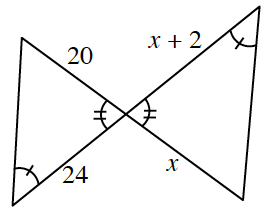### Home > INT1 > Chapter 7 > Lesson 7.1.3 > Problem7-41

7-41.

The triangles in the diagram at right are similar. Solve for $x$. Show all work.

When two triangles are similar, the ratios of corresponding sides are equal.
You could use a proportion.

$x = 10$